# Multiple Linear Regression

In this lesson, you will be extending your knowledge of simple linear regression, where you were predicting a quantitative response variable using a quantitative explanatory variable. That is, you were using an equation that looked like this:

\hat{y} = b_0 + b_1x_1

In this lesson, you will learn about multiple linear regression. In these cases, you will be using both quantitative and categorical x-variables to predict a quantitative response. That is, you will be creating equations that like this to predict your response:

\hat{y} = b_0 + b_1x_1 + b_2x_2 + b_3x_3 + b_4x_4

Furthermore, you will learn about how to assess problems that can happen in multiple linear regression, how to address these problems, and how to assess how well your model is performing. It turns out Rsquared can be used, but might be misleading. And, unfortunately, the correlation coefficient is only a measure of the linear relationship between two quantitative variables, so it will not be very useful in the multiple linear regression case.

A great additional resource is available here: Introduction to Statistical Learning the full text. This is an absolutely spectacular book for getting started with machine learning.

Specifically, Chapter 3 discusses many of the ideas in this lesson. The programming performed in the text is in R, but we will continue to use Python in this course.

If you want to fully grasp how the functions we will be using in Python work to fit multiple linear regression models, it is absolutely necessary to have a firm grasp of linear algebra. Though you can complete this class (and fit multiple linear regression models in python) without knowing linear algebra, linear algebra is useful for understanding why you do or do not obtain certain results from Python, as well as troubleshooting if something goes wrong with fitting your model.

You will see a few instances of strange output that you may obtain from multiple linear regression output as you work through this course. Two additional resources are listed below if you need a linear algebra refresher before you continue!

### Multiple Linear Regression Introduction

import numpy as np
import pandas as pd
import statsmodels.api as sms;

1. Using statsmodels, fit three individual simple linear regression models to predict price. You should have a model that uses area, another using bedrooms, and a final one using bathrooms. You will also want to use an intercept in each of your three models.

Use the results from each of your models to answer the first two quiz questions below.

df[‘intercept’] = 1
lm_bath = sms.OLS(df[‘price’], df[[‘intercept’, ‘bathrooms’]])
results_bath = lm_bath.fit()
results_bath.summary()

lm_bed = sms.OLS(df[‘price’], df[[‘intercept’, ‘bedrooms’]])
results_bed = lm_bed.fit()
results_bed.summary()

lm_area = sms.OLS(df[‘price’], df[[‘intercept’, ‘area’]])
results_area = lm_area.fit()
results_area.summary()

2. Now that you have looked at the results from the simple linear regression models, let’s try a multiple linear regression model using all three of these variables at the same time. You will still want an intercept in this model.

mlr = sms.OLS(df[‘price’], df[[‘intercept’, ‘area’, ‘bedrooms’, ‘bathrooms’]])
results_mlr = mlr.fit()
results_mlr.summary()

3. Along with using the areabedrooms, and bathrooms you might also want to use style to predict the price. Try adding this to your multiple linear regression model. What happens? Use the final quiz below to provide your answer.

mlr2 = sms.OLS(df[‘price’], df[[‘intercept’, ‘area’, ‘bedrooms’, ‘bathrooms’, ‘style’]])
results_mlr2 = mlr2.fit()
results_mlr2.summary()

X = df[[‘intercept’, ‘bathrooms’, ‘bedrooms’, ‘area’]]

y = df[‘price’]

np.dot(np.dot(np.linalg.inv(np.dot(X.transpose(),X)), X.transpose()),y)

The text noted at the end of the video is provided below.

How Do We Find the “Right” Coefficients in Multiple Linear Regression

In the simple linear regression section, you saw how we were interested in minimizing the squared distance between each actual data point and the predicted value from our model.

But in multiple linear regression, we are actually looking at points that live in not just a two dimensional space.

For a full derivation of how this works, this article provides a break down of the steps.

The takeaway for us is that we can find the optimal \beta estimates by calculating (X’X)^-X’y.

In the following video, you will use statsmodels to obtain the coefficients similar to in the last concept, but you will also solve for the coefficients using the equation above to show the results are not magic.

In this video, the coefficients were all positive. Therefore, we can interpret each coefficient as the predicted increase in the response for every one unit increase in the explanatory variable, holding all other variables in the model constant.

However, in general, coefficients might be positive or negative. Therefore, each coefficient is the predicted change in the response for every one unit increase in the explanatory variable, holding all other variables in the model constant.

This interpretation is very similar to what you saw in the last lesson with the simple addition of the phrase “holding all other variables constant” meaning only the variable attached to the coefficient changes, while all other variables stay the same.

The way that we add categorical variables into our multiple linear regression models is by using dummy variables. The most common way dummy variables are added is through 1, 0 encoding. In this encoding method, you create a new column for each level of a category (in this case A, B, or C). Then our new columns either hold a 1 or 0 depending on the presence of the level in the original column.When we add these dummy variables to our multiple linear regression models, we always drop one of the columns. The column you drop is called the baseline. The coefficients you obtain from the output of your multiple linear regression models are then an indication of how the encoded levels compare to the baseline level (the dropped level).

### The Math Behind Dummy Variables

In the last video, you were introduced to the idea the way that categorical variables will be changed to dummy variables in order to be added to your linear models.

Then, you will need to drop one of the dummy columns in order to make your matrices full rank.

If you remember back to the closed form solution for the coefficients in regression, we have \beta is estimated by (X’X)^-X’y.

In order to take the inverse of (X’X), the matrix X must be full rank. That is, all of the columns of X must be linearly independent.

If you do not drop one of the columns when creating the dummy variables, your solution is unstable and results from python are unreliable. You will see an example of what happens if you do not drop one of the dummy columns in the next concept.

The takeaway is…when you create dummy variables using 0, 1 encodings, you always need to drop one of the columns to make sure your matrices are full rank (and that your solutions are reliable from python).

The reason for this is linear algebra. Specifically, in order to invert matrices, a matrix must be full rank (that is all the columns need to be linearly independent). Therefore, you need to drop one of the dummy columns, to create linearly independent columns (and a full rank matrix).

### Dummy Variables

You saw in the earlier notebook that you weren’t able to directly add a categorical variable to your multiple linear regression model. In this notebook, you will get some practice adding dummy variables to your models and interpreting the output.

Let’s start by reading in the necessary libraries and data.

import numpy as np
import pandas as pd
import statsmodels.api as sm;
import matplotlib.pyplot as plt
%matplotlib inline

1. Use the pd.get_dummies documentation to assist you with obtaining dummy variables for the neighborhood column. Then use join to add the dummy variables to your dataframe, df, and store the joined results in df_new.

Fit a linear model using all three levels of neighborhood neighborhood to predict the price. Don’t forget an intercept.

neighborhood_dummies = pd.get_dummies(df[‘neighborhood’])
df_new = df.join(neighborhood_dummies)

df_new[‘intercept’] = 1
lm = sm.OLS(df_new[‘price’], df_new[[‘intercept’, ‘A’, ‘B’, ‘C’]])
results = lm.fit()
results.summary()

2. Now, fit an appropriate linear model for using neighborhood to predict the price of a home. Use neighborhood A as your baseline. Use your resulting model to answer the questions in Quiz 2 and Quiz 3 below.

lm2 = sm.OLS(df_new[‘price’], df_new[[‘intercept’, ‘B’, ‘C’]])
results2 = lm2.fit()
results2.summary()

3. Run the two cells below to look at the home prices for the A and C neighborhoods. Add neighborhood B. This creates a glimpse into the differences that you found in the previous linear model.

plt.hist(df_new.query(“C == 1”)[‘price’], alpha = 0.3, label = ‘C’);
plt.hist(df_new.query(“A == 1”)[‘price’], alpha = 0.3, label = ‘A’);

plt.legend();

4. Now, add dummy variables for the style of house, as well as neighborhood. Use ranch as the baseline for the style. Additionally, add bathrooms and bedrooms to your linear model. Don’t forget an intercept. Use the results of your linear model to answer the last two questions below. Home pricess are measured in dollars, and this dataset is not real.

type_dummies = pd.get_dummies(df[‘style’])
df_new = df_new.join(type_dummies)

lm3 = sm.OLS(df_new[‘price’], df_new[[‘intercept’, ‘B’, ‘C’, ‘lodge’, ‘victorian’, ‘bedrooms’, ‘bathrooms’]])
results3 = lm3.fit()
results3.summary()

Though python and R use 1, 0 encodings in the default functionality, there are many ways we might encode dummy variables. SAS is a common programming language that uses a different encoding than you have used so far. Being comfortable with the 1, 0 encodings is really all you need, but if you are curious about other encodings you can try out the quiz on the next page to see how this works.

### Model Assumptions And How To Address Each

Here are the five assumptions addressed in the previous video addressed in Introduction to Statistical Learning:

1. Non-linearity of the response-predictor relationships
2. Correlation of error terms
3. Non-constant Variance and Normally Distributed Errors
4. Outliers/ High leverage points
5. Collinearity

This text is a summary of how to identify if these problems exist, as well as how to address them. This is a common interview question asked by statisticians, but its practical importance is hit or miss depending on the purpose of your model. In the upcoming concepts, we will look more closely at specific points that I believe deserve more attention, but below you will see a more exhaustive introduction to each topic. Let’s take a closer look at each of these items.

#### Linearity

The assumption of linearity is that a linear model is the relationship that truly exists between your response and predictor variables. If this isn’t true, then your predictions will not be very accurate. Additionally, the linear relationships associated with your coefficients really aren’t useful either.

In order to assess if a linear relationship is reasonable, a plot of the residuals (y – \hat{y}) by the predicted values (\hat{y}) is often useful. If there are curvature patterns in this plot, it suggests that a linear model might not actually fit the data, and some other relationship exists between the predictor variables and response. There are many ways to create non-linear models (even using the linear model form), and you will be introduced to a few of these later in this lesson.

In the image at the bottom of this page, these are considered the biased models. Ideally, we want to see a random scatter of points like the top left residual plot in the image.

#### Correlated Errors

Correlated errors frequently occur when our data are collected over time (like in forecasting stock prices or interest rates in the future) or data are spatially related (like predicting flood or drought regions). We can often improve our predictions by using information from the past data points (for time) or the points nearby (for space).

The main problem with not accounting for correlated errors is that you can often use this correlation to your advantage to better predict future events or events spatially close to one another.

One of the most common ways to identify if you have correlated errors is based on the domain from which the data where collected. If you are unsure, there is a test known as a Durbin-Watson test that is commonly used to assess whether correlation of the errors is an issue. Then ARIMA or ARMA models are commonly implemented to use this correlation to make better predictions.

#### Non-constant Variance and Normally Distributed Errors

Non-constant variance is when the spread of your predicted values differs depending on which value you are trying to predict. This isn’t a huge problem in terms of predicting well. However, it does lead to confidence intervals and p-values that are inaccurate. Confidence intervals for the coefficients will be too wide for areas where the actual values are closer to the predicted values, but too narrow for areas where the actual values are more spread out from the predicted values.

Commonly, a log (or some other transformation of the response variable is done) in order to “get rid” of the non-constant variance. In order to choose the transformation, a Box-Cox is commonly used.

Non-constant variance can be assessed again using a plot of the residuals by the predicted values. In the image at the bottom of the page, non-constant variance is labeled as heteroscedastic. Ideally, we want an unbiased model with homoscedastic residuals (consistent across the range of values).

Though the text does not discuss normality of the residuals, this is an important assumption of regression if you are interested in creating reliable confidence intervals. More on this topic is provided here.

#### Outliers/Leverage Points

Outliers and leverage points are points that lie far away from the regular trends of your data. These points can have a large pull on your solution. In practice, these points might even be typos. If you are aggregating data from multiple sources, it is possible that some of the data values were carried over incorrectly or aggregated incorrectly.

Other times outliers are accurate and true data points, not necessarily measurement or data entry errors. In these cases, ‘fixing’ is more subjective. Often the strategy for working with these points is dependent on the goal of your analysis. Linear models using ordinary least squares, in particular, are not very robust. That is, large outliers may greatly change our results. There are techniques to combat against this – largely known as regularization techniques. These are beyond the scope of this class, but they are quickly discussed in the free version of the Machine Learning Nanodegree.

An entire course on regression is provided by Penn State, and they spend a particularly large amount of time discussing the topics of leverage points here.

#### Collinearity (Multi-collinearity)

Multicollinearity is when we have predictor variables that are correlated with one another. One of the main concerns of multicollinearity is that it can lead to coefficients being flipped from the direction we expect from simple linear regression.

One of the most common ways to identify multicollinearity is with bivariate plots or with variance inflation factors (or VIFs). This is a topic we will dive into in the next concept, so we won’t spend as much time on it here.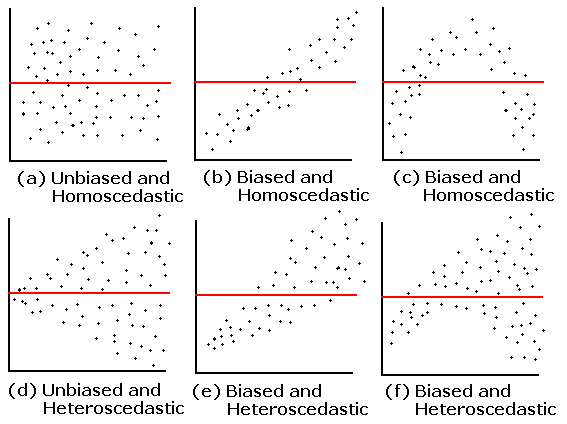### Multicollinearity & VIFs

import pandas as pd
import numpy as np
import seaborn as sns
from patsy import dmatrices
import statsmodels.api as sm;
from statsmodels.stats.outliers_influence import variance_inflation_factor
%matplotlib inline

1.Use seaborn to look at pairwise relationships for all of the quantitative, explanatory variables in the dataset by running the cell below. You might also try adding color (hue) for the house style or neighborhood. Use the plot to answer the first quiz questions below.

sns.pairplot(df[[‘bedrooms’, ‘bathrooms’, ‘area’]]);

2. Earlier, you fit linear models between each individual predictor variable and price, as well as using all of the variables and the price in a multiple linear regression model. Each of the individual models showed a positive relationship – that is, when bathrooms, bedrooms, or area increase, we predict the price of a home to increase.

Fit a linear model to predict a home price using bedroomsbathrooms, and area. Use the summary to answer the second quiz question below. Don’t forget an intercept.

df[‘intercept’] = 1

lm = sm.OLS(df[‘price’], df[[‘intercept’, ‘bathrooms’, ‘bedrooms’, ‘area’]])
results = lm.fit()
results.summary()

3. Calculate the VIFs for each variable in your model. Use quiz 3 below to provide insights about the results of your VIFs. Here is the helpful post again, in case you need it!

# get y and X dataframes based on this regression:
y, X = dmatrices(‘price ~ bedrooms + bathrooms + area’ , df, return_type=’dataframe’)

# For each X, calculate VIF and save in dataframe
vif = pd.DataFrame()
vif[“VIF Factor”] = [variance_inflation_factor(X.values, i) for i in range(X.shape)]
vif[“features”] = X.columns

vif

4. Remove bathrooms from your above model. Refit the multiple linear regression model and re-compute the VIFs. Use the final quiz below to provide insights about your results.

lm = sm.OLS(df[‘price’], df[[‘intercept’, ‘bedrooms’, ‘area’]])
results = lm.fit()
results.summary()

# get y and X dataframes based on this regression:
y, X = dmatrices(‘price ~ bedrooms + area’ , df, return_type=’dataframe’)

# For each X, calculate VIF and save in dataframe
vif = pd.DataFrame()
vif[“VIF Factor”] = [variance_inflation_factor(X.values, i) for i in range(X.shape)]
vif[“features”] = X.columns
vif

You saw in this video two different ways of identifying multicollinearity:

1. We can look at the correlation of each explanatory variable against each other explanatory variable (with a plot or the correlation coefficient).
2. We can look at VIFs for each variable, this calculation will be shown in more detail in the next video.

We saw that when x-variables are related to one another, we can have flipped relationships in our multiple linear regression models from what we would expect when looking at the bivariate linear regression relationships.

For more on VIFs and multicollinearity, here is the referenced post from the video on VIFs.

We would like x-variables to be related to the response, but not to be related to one another. When our x-variables are correlated with one another, this is known as multicollinearity. Multicollinearity has two potential negative impacts. As you saw in the previous example,

1. The expected relationships between your x-variables and the response may not hold when multicollinearity is present. That is, you may expect a positive relationship between the explanatory variables and the response (based on the bivariate relationships), but in the multiple linear regression case, it tuns out the relationship is negative.
2. Our hypothesis testing results may not be reliable. It turns out that having correlated explanatory variables means that our coefficient estimates are less stable. That is, standard deviations (often called standard errors) associated with your regression coefficients are quite large. Therefore, a particular variable might be useful for predicting the response, but because of the relationship it has with other x-variables, you will no longer see this association.

We have also looked at two different ways of identifying multicollinearity:

1. We can look at the correlation of each explanatory variable against each other explanatory variable (with a plot or the correlation coefficient).
2. We can look at VIFs for each variable, this calculation will be shown in more detail in the next video.

When VIFs are greater than 10, this suggests that multicollinearity is certainly a problem in your model. Some experts even suggest VIFs of greater than 5 can be problematic. In most cases, not just one VIF is high, rather many VIFs are high, as these are measures of how related variables are with one another.

The most common way of working with correlated explanatory variables in a multiple linear regression model, is simply to remove one of the variables that is most related to the other variables. Choosing an explanatory variable that you aren’t interested in, or isn’t as important to you, is a common choice.

### How to Identify Higher Order Terms?

Higher order terms in linear models are created when multiplying two or more x-variables by one another. Common higher order terms include quadratics (x_1^2) and cubics (x_1^3) , where an x-variable is multiplied by itself, as well as interactions (x_1x_2) , where two or more x-variables are multiplied by one another.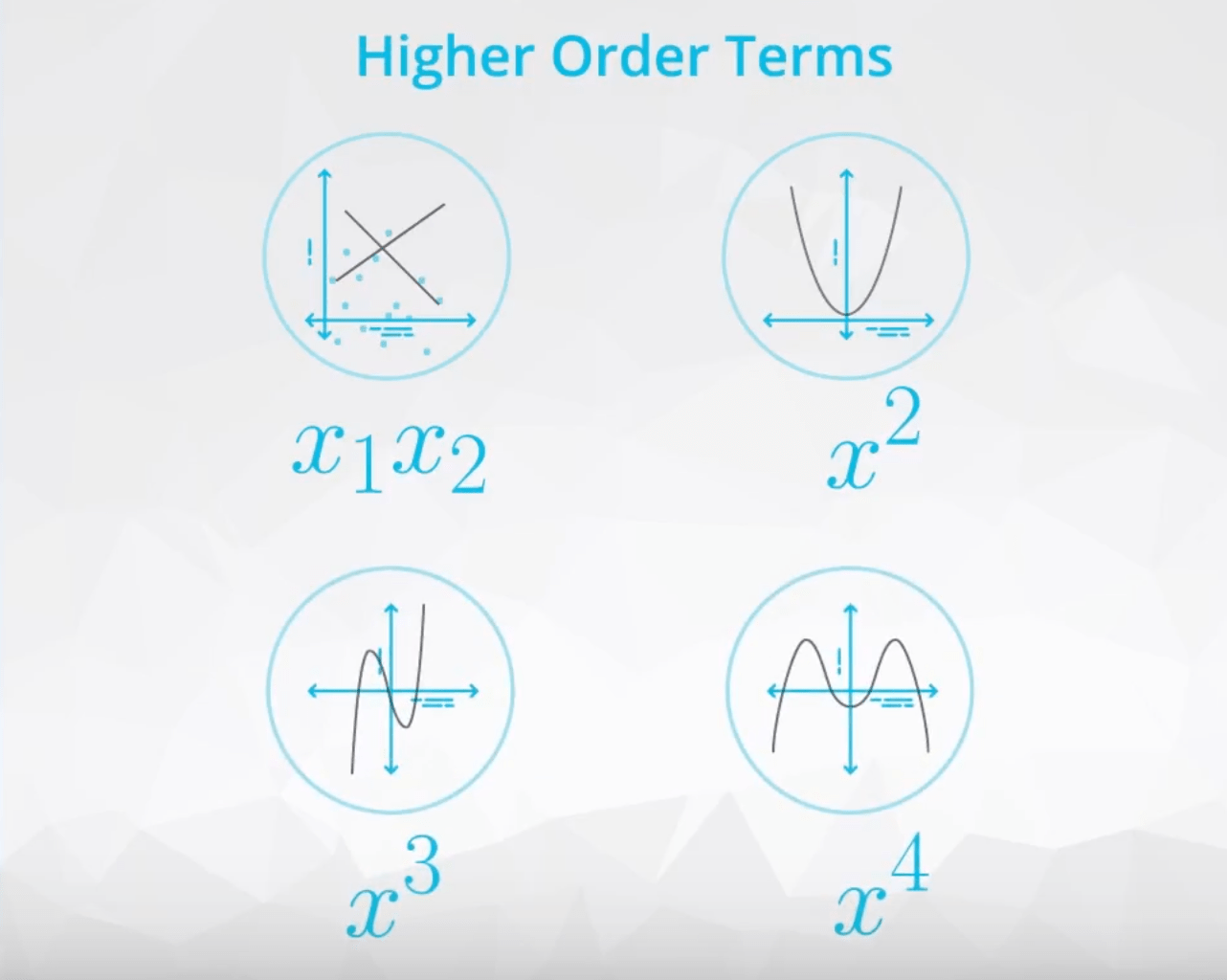In a model with no higher order terms, you might have an equation like:

\hat{y} = b_0 + b_1x_1 + b_2x_2

Then we might decide the linear model can be improved with higher order terms. The equation might change to:

\hat{y} = b_0 + b_1x_1 + b_2x_1^2 +b_3x_2 + b_4x_1x_2

Here, we have introduced a quadratic (b_2x_1^2) and an interaction (b_4x_1x_2) term into the model.

In general, these terms can help you fit more complex relationships in your data. However, they also takeaway from the ease of interpreting coefficients, as we have seen so far. You might be wondering: “How do identify if I need one of these higher order terms?”

When creating models with quadraticcubic, or even higher orders of a variable, we are essentially looking at how many curves there are in the relationship between the explanatory and response variables.

If there is one curve, like in the plot below, then you will want to add a quadratic. Clearly, we can see a line isn’t the best fit for this relationship.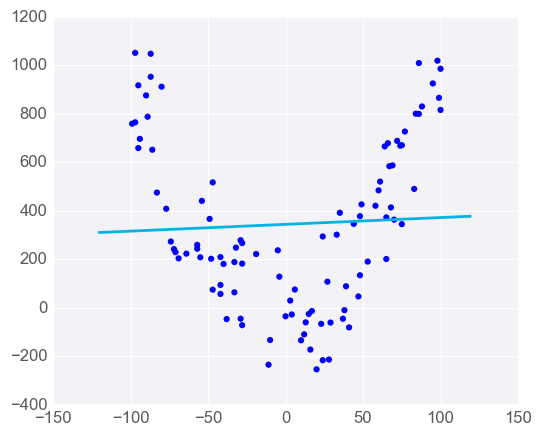Then, if we want to add a cubic relationship, it is because we see two curves in the relationship between the explanatory and response variable. An example of this is shown in the plot below.Diving into these relationships a little more closely and creating them in your linear models in python will be the focus in the upcoming videos.

### Interaction Terms

In the previous video, you were introduced to how you might go about interpreting interactions (or in being able to identify them.

Mathematically, an interaction is created by multiplying two variables by one another and adding this term to our linear regression model.The example from the previous video used area (x_1) and the neighborhood (x_2) of a home (either A or B) to predict the home price (y). At the top of the screen in the video, you might have noticed the equation for a linear model using these variables as:

\hat{y} = b_0 + b_1x_1 + b_2x_2

This example does not involve an interaction term, and this model is appropriate if the relationship of the variables looks like that in the plot below.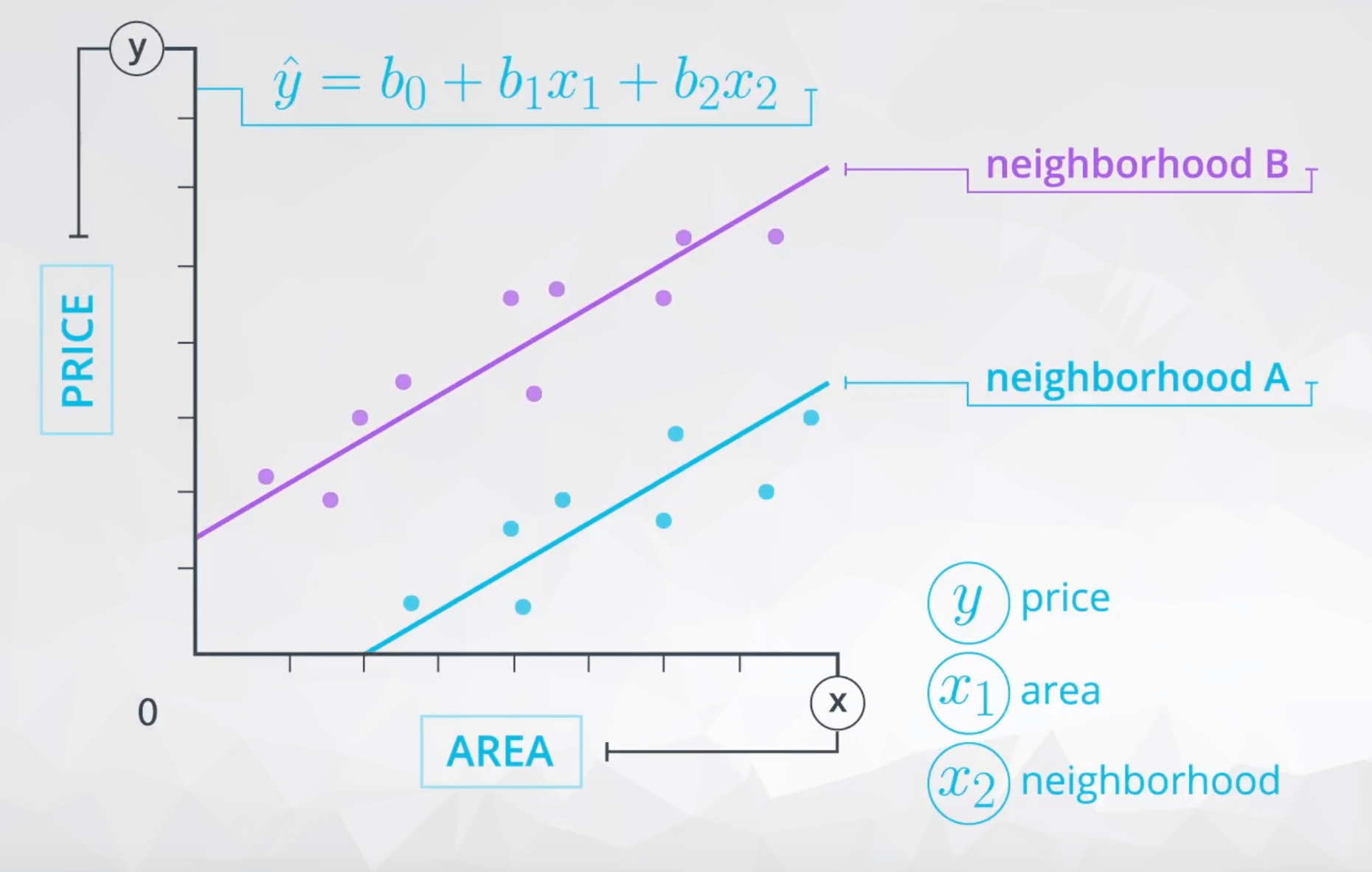where b_1 is the way we estimate the relationship between area and price, which in this model we believe to be the same regardless of the neighborhood.

Then b_2 is the difference in price depending on which neighborhood you are in, which is the verticaldistance between the two lines here: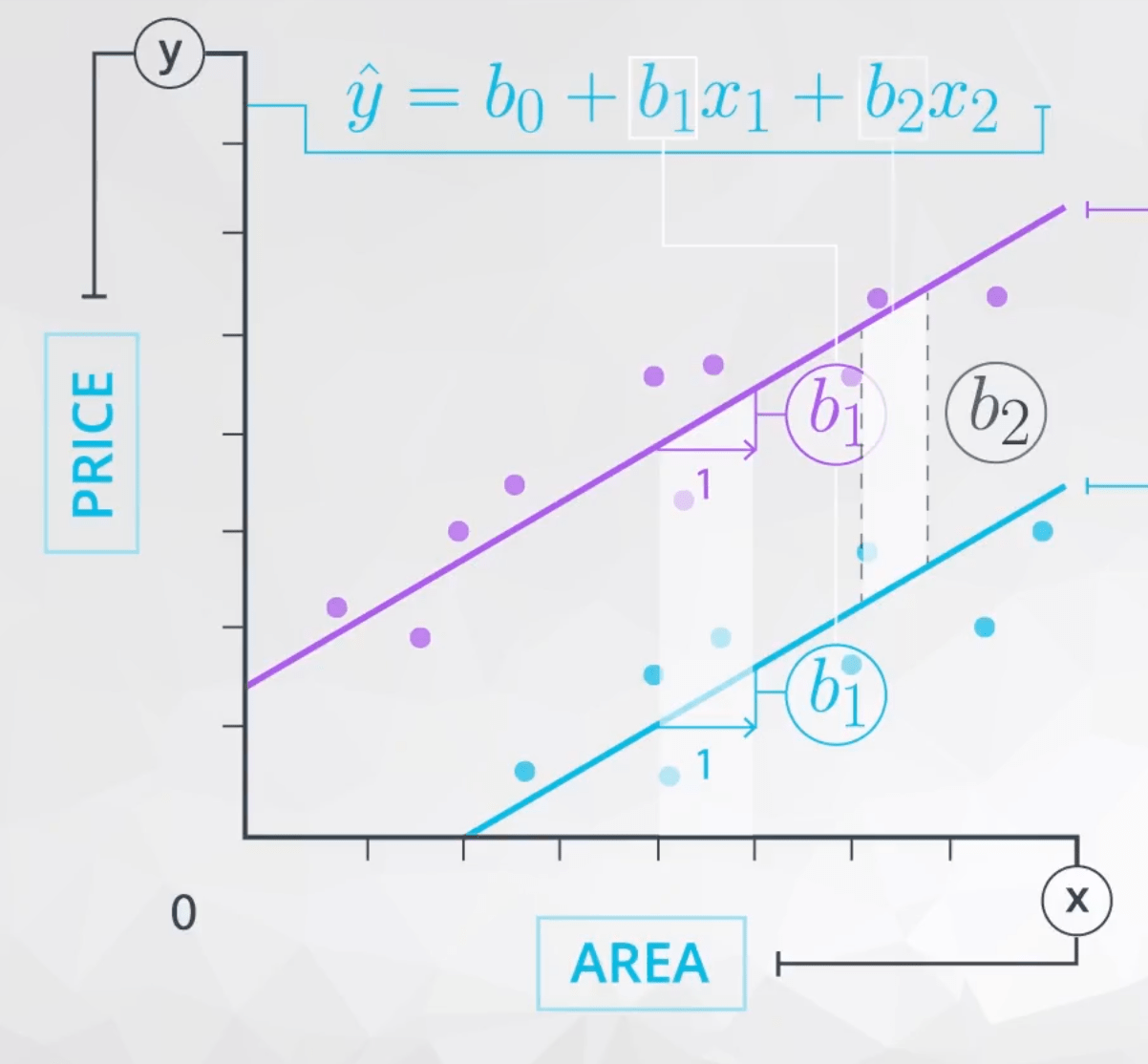Notice, here the way that area is related to price is the same regardless of neighborhood.

AND

The difference in price for the different neighborhoods is the same regardless of the area.

When these statements are true, we do not need an interaction term in our model. However, we need an interaction when the way that area is related to price is different depending on the neighborhood.

Mathematically, when the way area relates to price depends on the neighborhood, this suggests we should add an interaction. By adding the interaction, we allow the slopes of the line for each neighborhood to be different, as shown in the plot below. Here, we have added the interaction, and you can see this allows for a difference in these two slopes.These lines might even cross or grow apart quickly. Either of these would suggest an interaction is present between area and neighborhood in the way they related to the price.

### Model 1

1. For the first model, fit a model to predict price using neighborhoodstyle, and the area of the home. Use the output to match the correct values to the corresponding interpretation in quiz 1 below. Don’t forget an intercept! You will also need to build your dummy variables, and don’t forget to drop one of the columns when you are fitting your linear model. It may be easiest to connect your interpretations to the values in the first quiz by creating the baselines as neighborhood C and home style lodge.

# Adding the intercept, and creating our dummy variables
df[‘intercept’] = 1
df[[‘A’, ‘B’, ‘C’]] = pd.get_dummies(df[‘neighborhood’])
df[[‘lodge’, ‘ranch’, ‘victorian’]] = pd.get_dummies(df[‘style’])

lm = sm.OLS(df[‘price’], df[[‘intercept’, ‘A’, ‘B’, ‘ranch’, ‘victorian’, ‘area’]])
results = lm.fit()
results.summary()

df.groupby(‘style’).mean()[[‘price’, ‘area’]]

Turns out we can interpret all of the coefficients in this first model. Since there are no higher order terms, the dummy variables and quantitative variables are interpreted just as you have seen in previous concepts.

### Model 2

2. Now let’s try a second model for predicting price. This time, use area and area squared to predict price. Also use the style of the home. You will again need to use your dummy variables, and add an intercept to the model. Use the results of your model to answer quiz 2.

# Adding the intercept, and creating our dummy variables
df[‘area_squared’] = df[‘area’]*df[‘area’]

lm2 = sm.OLS(df[‘price’], df[[‘intercept’, ‘area’, ‘area_squared’, ‘ranch’, ‘victorian’]])
results2 = lm2.fit()
results2.summary()

With the higher order term, the coefficients associated with area and area squared are not easily interpretable. However, coefficients that are not associated with the higher order terms are still interpretable in the way you did earlier.

A best model might only include the area, and a dummy variable for neighborhood B vs. the other neighborhoods.

Judging by the first results from the two models you built, the best would likely involve only these two variables, as it would be simplified, while still predicting well.

### Recap

1. You learned how to build a multiple linear regression model in python, which was actually very similar to what you did in the last lesson on simple linear regression.
2. You learned how to encode dummy variables, and interpret the coefficients attached to each.
3. You learned about higher order terms, and how this impacts your ability to interpret coefficients.
4. You learned how to identify what it would mean for an interaction to be needed in a multiple linear regression model, as well as how to identify other higher order terms. But again, these do make interpreting coefficients directly less of a priority, and move your model towards one that, rather, aims to predict better at the expense of interpretation.
5. You learned about the model assumptions, and we took a closer look at multicollinearity. You learned about variance inflation factors, and how multicollinearity impacts the model coefficients and standard errors.